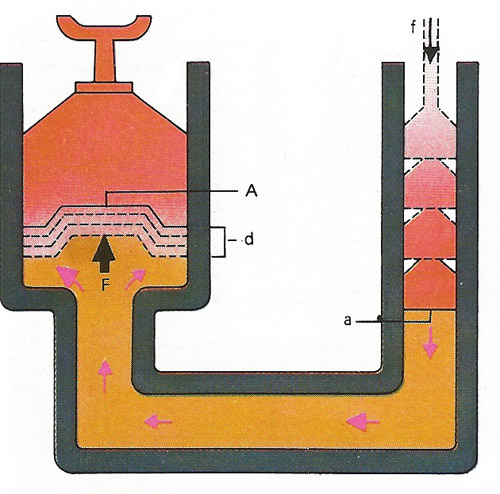A# hydrodynamicsHydraulic lift.

Hydrodynamics is the branch of fluid dynamics dealing with the motion, energy, and pressures of liquids in motion. A mathematical treatment of ideal frictionless and incompressible fluids flowing around given boundaries is coupled with an empirical approach in order to solve practical problems.

Motion changes the pressure within a fluid, and this can be difficult to predict accurately. The flow can be either smooth (streamlined) or turbulent, when the fluid is broken up into eddies; it is then harder to calculate the pressure at different points within the fluid.

## Hydraulic lift

A hydraulic lift (see diagram) works on the principle that an incompressible liquid transmits pressure equally in all directions. The small force f acting on area a produces pressure f/a which is converted at the much larger area A to the correspondingly larger force F so that F/A = f/a. The larger force can be used to move great weights. But the weight moves through only the small distance d.